## How about a date (function)?

Feb 14

Written by: Charles Flock
2/14/2011 9:33 PMWe added 3 new date functions to XLeratorDB/financial that should make date calculations and date manipulations much easier and more EXCEL-like, and show you how to use them to calculate things like date of Easter.
Long-time users of EXCEL will be familiar with its DATE function. It’s simple, intuitive, and allows for quite complex date calculations in EXCEL. Of course, the one thing that it does not do, technically, is calculate a date. It returns an integer that represents a date.
We wanted the same clean, uncomplicated function in SQL Server. We have implemented the DATEINT function as an analogue to the EXCEL DATE function. If we could have, we would have called it DATE, but DATE is a reserved word in .NET, so we settled on DATEINT.
The function is very straightforward.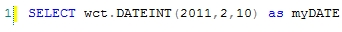This produces the following result.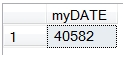We have also implemented the CALCDATE function, which has exactly the same calling structure as the DATEINT function, but returns a datetime value rather than an int value.This produces the following result.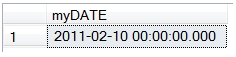There is also a DATEFLOAT function, which has the same calling structure as DATEINT, but returns a float value rather than an int value.
Before delving too much further into some of the ways that you can use these new functions, we need to talk about the differences in date representation among .NET, SQL Server, and EXCEL. Let’s start with SQL Server.
The integer representation of a date in SQL Server, basically, is the number of days since 01-Jan-1900. This means that 01-Jan-1900 has an integer value of 0 and 10-Feb-2010 has an integer value of 40582.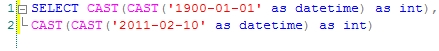This produces the following result.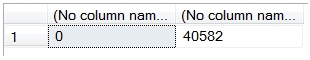If we go to EXCEL, however, we will get a different result.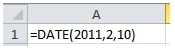This produces the following result.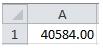Excel’s value is 2 days greater than SQL Server’s. There are 2 reasons for this. First, EXCEL treats 01-Jan-1900 as 1, so it seems like EXCEL is calculating the number of days from 31-Dec-1899. Second, EXCEL incorrectly assumes that 1900 is a leap year. Oops! Starting from 01-Mar-1900 the integer value of a date in EXCEL is going to be 2 more than integer value of a date in SQL Server.
Just to make things even more confusing, the .NET integer representation of a date is the same as EXCEL’s, except for dates prior to 01-Mar-1900, since .NET understands that 1900 is not a leap year.
This actually presented us with an interesting challenge, especially since we like to be able to simply copy formulae out of an EXCEL spreadsheet and paste into SQL Server and have it produce the same results. For example, here’s a handy EXCEL formula for calculating the date of Easter.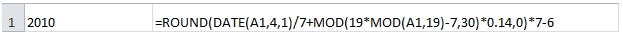The calculation begins by setting the date equal to April 1st in the year entered in A1. Of course, since EXCEL doesn’t store dates, it’s really setting it to a number. However that number will be 2 days greater than the number in SQL Server, so entering the same formula will produce an inaccurate result.
Even though we like the ability to be able to copy EXCEL formulae more or less directly into SQL Server, we felt that it would be just too confusing to use the EXCEL/.NET integer representations. So, our new date functions have integer representations consistent with SQL Server’s.
Thus, we would take the EXCEL formula above and represent it in the following SQL which will calculate Easter for the years 2010 through 2050.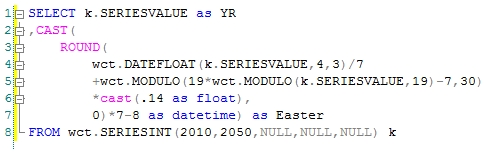The produces the following result.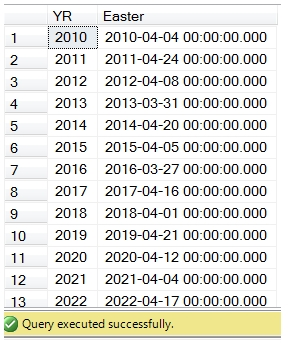EXCEL produces the identical result.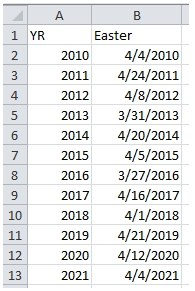Notice, though, that we had to change the date value by 2 days in SQL.
You might also notice that we used the MODULO function (which is the equivalent of the EXCEL MOD function) instead of the SQL Server modulo symbol, %. This is because the SQL Server modulo function is really a remainder function. The SQL Server documentation uses the terms interchangeably, which is really confusing, but the SQL Server modulo function calculates a remainder. Modulo and remainder for positive integers are the same, but for negative numbers they are not. Many of the date calculations that I am used to doing in EXCEL, require a true modulo function and the use of the SQL Server modulo function may produce inaccurate results.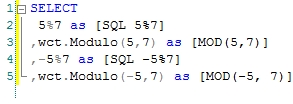This produces the following result.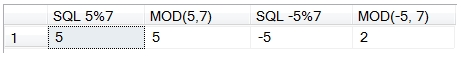Like the EXCEL date function, these date functions are very forgiving. Here’s an example where we have a table containing the year and month and we want to calculate the last calendar day of the month from the table. Here’s how we would do using CALCDATE.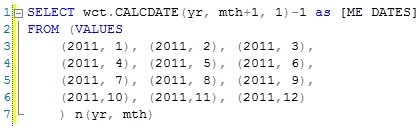This produces the following result.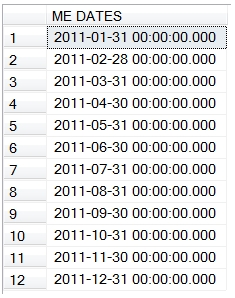We could have achieved the same result without using the CALCDATE function by entering this SQL.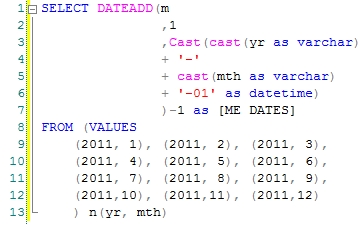As you can see, we had to convert the year and month to strings, concatenate the strings with dashes and append a 1 to the string, cast the string as datetime, use the DATEADD function to add 1 month, and then subtract one day. This is exactly the same logic that was used by selecting CALCDATE(yr, mth+1, 1) -1, but a lot more to key in and a lot harder to follow.
What if we wanted to change the calculation to be the last Friday of the month? Let’s look at how we would do that in EXCEL.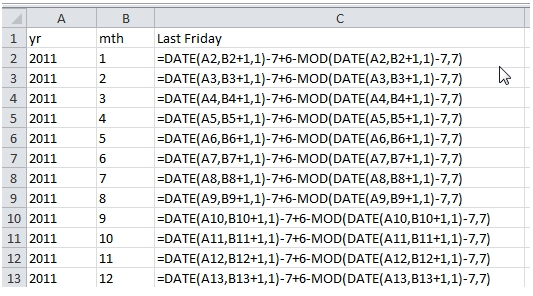This produces the following result.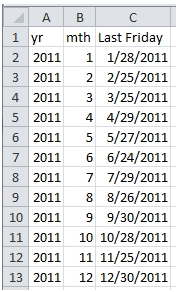With the DATEINT function, it’s pretty easy to translate this formula from EXCEL to SQL Server. We just need to remember that the integer representation of dates in EXCEL is 2 greater than in SQL Server. So we need to add 2 as we go into the formula and subtract 2 as we come out. This is what the SQL looks like.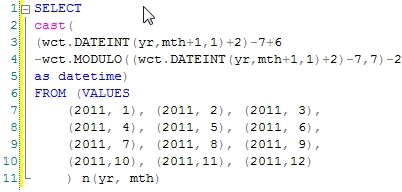This produces the following result.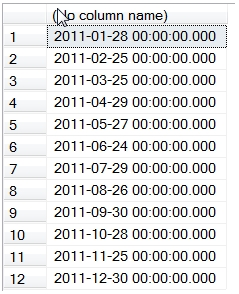I really like the simplicity of being able to take the function right out of EXCEL and paste it into SQL server and just tweak it a little bit. This is the same calculation in native SQL.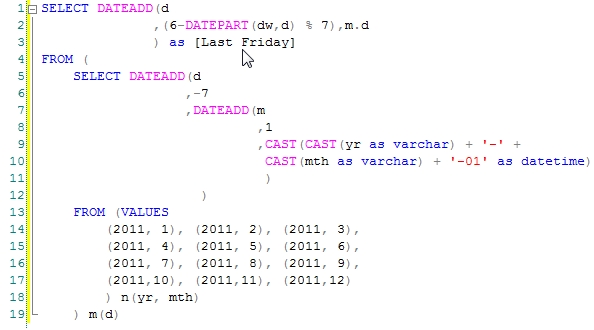This produces the following result.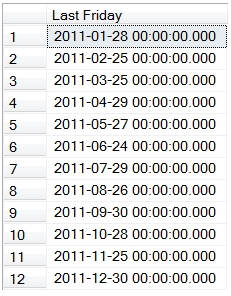While we can do the calculation in native SQL, it’s more complicated and it’s harder to go straight from the EXCEL formula to the SQL. In fact, I elected to use the derived table (m), because there were so many levels of data nested functions, that I was losing track of where to put the commas and parenthesis, even with Intellisense. This means the debugging is that much harder.
We also used the built-in modulo function (%), because we structured the query in such a way that we would always be passing a positive integer into the function.

I like having a date function that works like EXCELS. It lets me continue to model things in EXCEL and makes the transition from my EXCEL model to SQL simple and straightforward. What do you think?

Tags:
Categories:
Location: BlogsThe WestClinTech Blog

## Search Blogs

 KeywordsPhrase

## Blog Archives

Archive
 < September 2023 >
SunMonTueWedThuFriSat
272829303112
3456789
10111213141516
17181920212223
24252627282930
1234567
Monthly
Go

### SupportCopyright 2008-2023 Westclintech LLC         Privacy Policy        Terms of Service